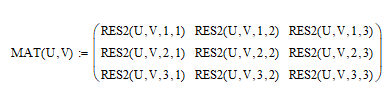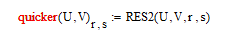cancel
Showing results for
Did you mean:
cancel
Showing results for
Did you mean:

SOLVED
Highlighted

Symbolic matrix construction

I try to create a symbolic matrix (in my example, the matrix RES  ).

The problem is that I cannot have the result in the form of a matrix, the only way to obtain what I want is to say that RES is not a matrix and is depending on two others variables corresponding to the indexes of the "matrix" (RES2 solution in the attached file question.xmcd). However, I need to use this result after and I need to have the result in the form of matrix.

How can I do to obtain my result in the form of a matrix ?

Thanks !

Tags (2)
1 ACCEPTED SOLUTION

Accepted Solutions

Re: Symbolic matrix construction

Hi,You cannot index a function at its definition.  Mathcad is looking for the name of a variable to index.

The second function definition does work as it does a number of summations that return a single value as a result of the function.

You can define a matrix that uses this function.  Is this what you are after?Warning.  by default Mathcad indexes matrices from 0,0 unless you redefine ORIGIN worksheet variable.

Cheers

Terry

9 REPLIES 9

Re: Symbolic matrix construction

Hi,You cannot index a function at its definition.  Mathcad is looking for the name of a variable to index.

The second function definition does work as it does a number of summations that return a single value as a result of the function.

You can define a matrix that uses this function.  Is this what you are after?Warning.  by default Mathcad indexes matrices from 0,0 unless you redefine ORIGIN worksheet variable.

Cheers

Terry

Re: Symbolic matrix construction

Thanks for your response !

Re: Symbolic matrix construction

Hi,

The answer would not be complete now we know the intent.

You can define the matrix with a quicker notation using the values of r and s you defined.

In this case a name is used in the definition so you can use indexes

Remember by default matrices start at index zero.Cheers

Terry

Re: Symbolic matrix construction

Hi,

This is exactly what I wanted, thanks !

But I still have a question, if I want to keep U and V as symbolic variable, the only way to obtain the matrix is to create each component "manually" as in your previous response ?

Thanks

JUF

Re: Symbolic matrix construction

Hi,

Not sure I understand your question.

U and V are variables in scope for the function RES2 definition.

V is also a variable in scope for function MAT2 definition.

I don't want to call them symbolics as this has another description in symbolic arithmetic.

U and V in definition for RES2 are set to  the values 10 and 31 only in the definition of the final matrix in one statement.Re: Symbolic matrix construction

Hi,

I want to have this :where MAT(U,V) is a matrix (of function) and U and V are variables in scope for the MAT function. After, I will used this matrix for different values of U and V but for now I want keep U and V as vrariables.

My question is : can I do something like the print screen below?Or do I need to define each component of the matrix MAT as it's done in the previous printscreen ?

Re: Symbolic matrix construction

(There is the reply with printscreens, sorry)

Hi,

I want to have this :where MAT(U,V) is a matrix (of function) and U and V are variables in scope for the MAT function. After, I will used this matrix for different values of U and V but for now I want keep U and V as vrariables.

My question is : can I do something like the print screen below?Or do I need to define each component of the matrix MAT as it's done in the previous printscreen ?

Re: Symbolic matrix construction

Hi,

You cannot do this as Mathcad will not let you define a function with index values.You either do as in your matrix method which gets cumbersome with big matrices..Or use a small program to get around it.Cheers

Terry

Re: Symbolic matrix construction

Hi,

The solution with the for loop works perfectly  !

Thanks a lot Terry, It's really helpful.

Cheers,

Julian